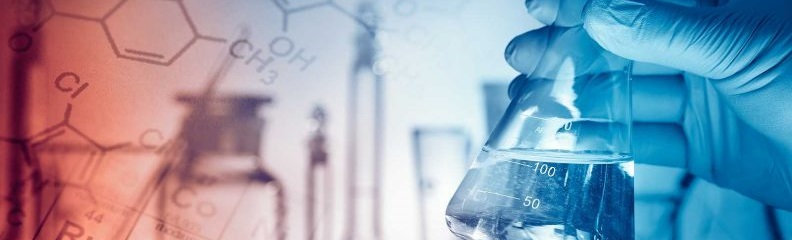# KS LearningMaths Platinum Certificate

### Maths Platinum Certificate Worksheets

The Maths Platinum Certificate is the fourth in a series of four certificates produced jointly by KS Learning and Porridge and Rice. The four certificates - Bronze, Silver, Gold, and Platinum - cover the AQA IGCSE Maths syllabus in four convenient sections and are designed to be taken in order before the IGCSE.

• P01 Quadratic equations, curves and inequalities

• Content
• P01.1 Quadratic functions and other graphs
• P01.2 Solving equations graphically

• 1) question 1:
1. 111
2. 222
3. 333
4. 444

• 2) question 2:
1. 111
2. 222
3. 333
4. 444

• 3) question 3:
1. 111
2. 222
3. 333
4. 444

• Content
• P02.1 Linear inequalities
• P02.3 Graphical inequalities

• 1) question 1:
1. 111
2. 222
3. 333
4. 444

• 2) question 2:
1. 111
2. 222
3. 333
4. 444

• 3) question 3:
1. 111
2. 222
3. 333
4. 444
• P03 Functions and function notation

• Content
• P03.1 Domain and range
• P03.2 Inverse functions
• P03.3 Compostite functions

• 1) question 1:
1. 111
2. 222
3. 333
4. 444

• 2) question 2:
1. 111
2. 222
3. 333
4. 444

• 3) question 3:
1. 111
2. 222
3. 333
4. 444
• P04 Further trigonometry

• Content
• P04.1 Translations and reflections
• P04.2 Rotations and enlargements

• 1) question 1:
1. 111
2. 222
3. 333
4. 444

• 2) question 2:
1. 111
2. 222
3. 333
4. 444

• 3) question 3:
1. 111
2. 222
3. 333
4. 444
• P05 Graphs and transformations

• Content
• P05.1 Using vectors
• P05.2 Magnitude of vectors

• 1) question 1:
1. 111
2. 222
3. 333
4. 444

• 2) question 2:
1. 111
2. 222
3. 333
4. 444

• 3) question 3:
1. 111
2. 222
3. 333
4. 444
• P06 Vectors

• Content
• P06.2 Turning points
• P06.3 Motion of a particle

• 1) question 1:
1. 111
2. 222
3. 333
4. 444

• 2) question 2:
1. 111
2. 222
3. 333
4. 444

• 3) question 3:
1. 111
2. 222
3. 333
4. 444
• P07 Calculus

• Content
• P07.1 Calculating probabilities
• P07.2 P(not happening)

• 1) question 1:
1. 111
2. 222
3. 333
4. 444

• 2) question 2:
1. 111
2. 222
3. 333
4. 444

• 3) question 3:
1. 111
2. 222
3. 333
4. 444
• P08 Revision Worksheet

• 1) question 1:
1. 111
2. 222
3. 333
4. 444

• 2) question 2:
1. 111
2. 222
3. 333
4. 444

• 3) question 3:
1. 111
2. 222
3. 333
4. 444
• P09 Probability

• Content
• P07.1 Calculating probabilities
• P07.2 P(not happening)

• 1) question 1:
1. 111
2. 222
3. 333
4. 444

• 2) question 2:
1. 111
2. 222
3. 333
4. 444

• 3) question 3:
1. 111
2. 222
3. 333
4. 444
• P10 Revision Worksheet

• 1) question 1:
1. 111
2. 222
3. 333
4. 444

• 2) question 2:
1. 111
2. 222
3. 333
4. 444

• 3) question 3:
1. 111
2. 222
3. 333
4. 444
Sites of Interest

### Confidence

A good tutor can build the confidence of a learner enabling subject success

### Skills

A private tutor can improve the skills a pupil needs to master a subject

### Progress

Regular tutoring can drive progress and better results in school subjects

### Support

Support can help students and parents make the right academic decisions